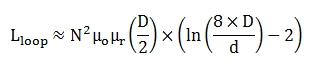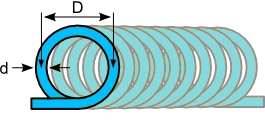# Coil Inductance Calculator

The coil inductance formula is used to calculate the inductance of chokes, transformers and similar structures.

mm
mm

#### Result

HClick here to view image

Where,

μo=4*3.142*1e-7

N - Number of Turns

D - Loop Diameter

d - Wire Diameter

μR - Relative Permeability